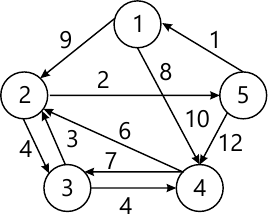MORE IN Design and Analysis of Algorithms
SPPU Information Technology (Semester 6)
Design and Analysis of Algorithms
December 2015
Total marks: --
Total time: --
INSTRUCTIONS
(1) Assume appropriate data and state your reasons
(2) Marks are given to the right of every question
(3) Draw neat diagrams wherever necessary

Solve any one question from Q1 and Q2
1 (a) Solve following recurrence relation:
T (n) = T(n/2) + 1
T(1) = 1.
5 M
1 (b) Analyze merge sort and find time complexity of merge sort.
5 M

2 (a) Write an algorithm to solve 'Tower of Hanoi' problem.
5 M
2 (b) Consider following instance for simple knapsack problem. Find the solution using greedy method.
N = 8
P = { 11, 21, 31, 33, 43, 53, 55, 65}
W = {1, 11, 21, 23, 33, 43, 45, 55}
M = 110
5 M

Solve any one question from Q3 and Q4
3 (a) Write Prim's algorithm to find minimum spanning tree.
5 M
3 (b) What is Principle of optimality? Differentiate between greedy and dynamic method.
5 M

4 (a) Write Dijkstra's algorithm to find all pairs shortest path.
5 M
4 (b) Write short note on: Proof by counterexample.
5 M

Solve any one question from Q5 and Q6
5 (a) Write an algorithm to find Hamiltonian path using backtracking method.
8 M
5 (b) Differentiate between backtracking and branch and bound. Draw state space tree for given sum of subset problem:
Set of elements = {3, 5, 6, 7} and d = 15
8 M

6 (a) What is backtracking? Write general recursive algorithm for backtracking.
8 M
6 (b) Discuss and analyze problem of graph coloring using backtracking with the help of example.
8 M

Solve any one question from Q7 and Q8
7 (a) Describe in brief the general strategy used in branch and bound method. Write general algorithm for Branch and Bound Method.
10 M
7 (b) Consider 0/1 Knapsack instance n = 4 with capacity 10 kg. such that
Find maximum profit using Least Cost branch and bound (LCBB) method. Use fixed size formation for state space tree.
 Item Profit (in Rs.) Weight (in Kg) 1 40 4 2 42 7 3 20 5 4 12 3
8 M

8 What is travelling salesman problem? Find the solution of following travelling salesman problem using branch and bound method.18 M

Solve any one question from Q9 and Q10
9 (a) Prove that vertex cover problem is NP complete.
8 M
9 (b) Explain in detail models for parallel computing.
8 M

10 (a) Explain : NP-Hard, NP-Complete, Decision Problem and Polynomial Time Algorithm.
8 M
10 (b) Write an algorithm for pointer doubling problem. What is the time complexity of this algorithm?
8 M

More question papers from Design and Analysis of Algorithms